# Arithmetic

## 一、题目要求及实现情况

• 输入
• 参数控制生成的题目个数 [√]

Myapp.exe -n 10

• 参数控制题目中操作数的范围：自然数[√]、真分数[x]、真分数分母[x]

Myapp.exe -r 10

• 生成
• 生成的题目
• 操作数：整数[√]、真分数[x]
• 符号：四则运算符[√]、空格分隔符[√]、等号[√]、左右括号[x][部分实现]
• 一题中运算符个数不超过三个 [√]
• 计算过程中不出现负数 [√]
• 一次性生成的题目不含重复的 [√]
• 能一次性生成一万道题 [√]
• 评卷
• 一次性生成参数要求数量题目，控制台处做一题评一题，最终汇总答题情况 [√]

• 对给定的题目文本和答案文本，评卷并输出总对错情况 [√]

Myapp.exe -e <exercisefile>.txt -a <answerfile>.txt

• 表现/输出
• 题目
• 生成的题目输出为 .txt 文本 [√]

• 题目文本格式为： [√]

1、四则运算题目1:

2、四则运算题目2:

• 答案
• 生成的答案输出为 .txt 文本 [√]

• 答案文本格式为： [√]

1、答案1

2、答案2

• 评卷
• 生成的评卷情况输出为 .txt 文本 [√]

• 评卷情况文本格式为： [√]

Correct:5(1,3,5,7,9)

Wrong:5(2,4,5,6,8)

[其中“:”后面的数字5表示对/错的题目的数量，括号内的是对/错题目的编号。为简单起见，假设输入的题目都是按照顺序编号的符合规范的题目。]

## 二、设计及具体实现

### 2、程序结构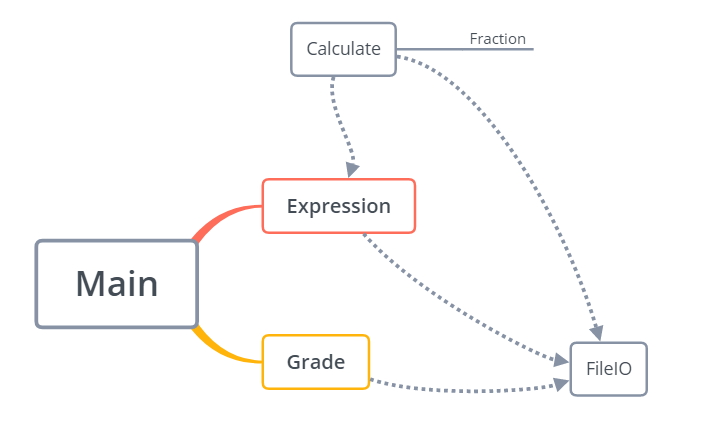### 3、代码说明

#### 项目共有6个类：Calculate,Expression, FileIO,Fraction,Grade ,Save

• Calculate 类通过将中缀表达式转化为后缀表达式，再求值。

• 中缀转后缀时，采用一个队列和一个栈，数字输入到队列，运算符压入栈。在压入栈时，检查栈顶元素的优先级，要保证压入栈的元素的优先级最高。若栈顶元素大于等于想压入栈的元素，则把栈顶元素弹出，继续检查，直到被压入栈的元素的优先级是最高的。遇到"("先入栈，遇到")"把"("之后的元素弹出到队列，括号不输出到队列。

• 后缀表达式求值时，遇到数字就压入栈，遇到运算符就弹出两个数字进行运算。因为最后的结果是分数，所以从一开始运算就把运算数全部转换为分数进行计算最后化简。

• 主要代码:

public Fraction calculate(Queue<String> queue) {//考虑分数的计算，直接把数字转化为fraction对象，再压入fraction栈
Stack<Fraction> fracStack = new Stack<>();

String str = "";//中间结果字符串
Save.string = "";//每一次操作开始就把string置为空

while (!queue.isEmpty() ) {

// 从队列中出队
String s = queue.remove();
// Save.save(s);//保存结果

// 如果是数字，就压入栈中
if (isDigital(s.charAt(0))) {
//parseInt(String s): 返回用十进制参数表示的整数值。
Fraction f = new Fraction(Integer.parseInt(s));
fracStack.push(f);
//   System.out.println("压入栈的f："+f.getNumerator()+"/"+f.getDenominator());

// 如果是运算符，就从栈中弹出两个元素
} else if (isOperator(s.charAt(0))) {
char c = s.charAt(0);

// System.out.println("当前的运算符"+c);
Fraction f = fracStack.pop();//操作数
Fraction f1 = new Fraction(f.getNumerator(),f.getDenominator());
f = fracStack.pop();//被操作数
Fraction f2 = new Fraction(f.getNumerator(),f.getDenominator());
/*
* 表达式合法性检验
* */
if ( c == '-' ) {
f = f2.sub(f1);//若结果为负，break；返回-1，用以标记表达式不合法
f.Appointment();//简单约分
if (f.getDenominator() < 0 || f.getNumerator() < 0  ) {//分母小于零出现负数
fracStack.push(new Fraction(100000));
break;

}
}
if ( c == '/'){
f2.Appointment();
if(f1.getDenominator()==0 || f1.getNumerator() ==0){//如果分数为0
fracStack.push(new Fraction(100000));
break;
}
}
/*
* 栈顶元素的四则运算
* */
switch (c) {
case '+': {
break;
}
case '-': {
fracStack.push(f2.sub(f1));
break;
}
case '*':
fracStack.push(f2.muti(f1));
break;
}
case '/': {
fracStack.push(f2.div(f1));
break;
}
}//switch case 结束的地方

/*
* 用来查重*/
str += fracStack.peek().getNumerator()
+"/"+fracStack.peek().getDenominator()+" ";///计算中间结果
}
}

Save.save(str);//保存中间结果
if(fracStack.isEmpty() == true || fracStack.peek().getNumerator() == 100000){//注意分子的值
Fraction f = new Fraction(100000);//不合法的式子
return f;
}else
return  fracStack.pop();
}

• Expression

• 共包含两个方法：

• generateExp(Integer limit)

运算数和运算符是随机生成的，每一次循环生成一个运算数和一个运算符，limit控制循环的次数，即运算符的数目。

• legalExp (Integer number,Integer limit)

在这个方法中对不合法和重复的式子进行剔除，不合法的情况有分母为零，出现负数。在调用Calculate方法计算的时候，把含有以上两种情况的式子都会返回100000，根据这个信息判断式子是否合法。查重时，通过比对式子和已生成的中间结果，如果重复则重新生成。把符合条件的式子写入到Expression文件中，答案写在Answer文件中。

• 主要代码：

    public void legalExp (Integer number,Integer limit) throws IOException {
/* 生成合法未重复的表达式，number表示题目数量,limit表示运算数范围*/
int j = 1;//控制题目生成的数量,从1开始

String str1 = "";
String str2 = "";//存放中间结果
String str3 = "";//算术表达式，写入Expression.txt

HashMap<String, Integer> answers = new HashMap<String, Integer>();

FileIO writer = new FileIO();//输入流对象
Expression exp = new Expression();//表达式对象

do {
str1 = exp.generateExp(limit) + "= ";//获得原始表达式
Calculate cal = new Calculate();
Fraction f = cal.outcome(str1);//计算结果，未化简

if (f.getNumerator() == 100000) {
// //剔除表达不合法的算术表达式
//   System.out.println("表达式出错");
continue;
}

str2 = f.transferFraction(f);//最终结果，已经化简

/*查重开始
存放值的时候，如果先检测下碰撞，如果有碰撞，则break 跳出do while 循环
* 如果没有碰撞，则存进去
通过比对中间结果是否相同,运算数在10以内，生成题目的上限是55778道题目，
随着运算数范围的缩小，上限更小
* */

//System.out.println("key碰撞");
continue;
}else{
// System.out.print("NO."+j+" "+str1 + "\n");//输出合法且没有重复的式子
System.out.printf("NO.%4d      %s%n",j,str1);//格式化输出

str3 += j+"."+"四则运算题目"+j+"    "+str1+"\n";
str4 += j+"."+"答案"+j+"    "+str2+"\n";
j++;
}

} while (j <= number);
System.out.println("表达式生成完毕");

writer.fileWrite(str3, Paths.get("textFile/Expression.txt"));//整个字符串

}

• Fraction

• 这个类是实现分数运算的核心类，封装相关的方法：构造分数函数，四则运算等

• 主要代码

      public Fraction( int numerator,int denominator) {
super();
this.denominator = denominator;
this.numerator = numerator;
}
// 构建一个可化简为整数的分数
public Fraction(int numerator) {
this.denominator = 1;
this.numerator = numerator;
}


java
// 加法运算
int a = r.getNumerator();// 获得分子
int b = r.getDenominator();// 获得分母
int newNumerator = numerator * b + denominator * a;
int newDenominator = denominator * b;
Fraction result = new Fraction(newNumerator,newDenominator);
return result;
}

// 减法运算
public Fraction sub(Fraction r) {
int a = r.getNumerator();// 获得分子
int b = r.getDenominator();// 获得分母
int newNumerator = numerator * b - denominator * a;
int newDenominator = denominator * b;
Fraction result = new Fraction(newNumerator,newDenominator);
return result;
}

// 分数的乘法运算
public Fraction muti(Fraction r) { // 乘法运算
int a = r.getNumerator();// 获得分子
int b = r.getDenominator();// 获得分母
int newNumerator = numerator * a;
int newDenominator = denominator * b;
Fraction result = new Fraction(newNumerator,newDenominator);
return result;
}

// 分数除法运算
public Fraction div(Fraction r) {
int a = r.getNumerator();// 获得分子
int b = r.getDenominator();// 获得分母
int newNumerator = numerator * b;
int newDenominator = denominator * a;
Fraction result = new Fraction(newNumerator,newDenominator);
return result;
}


• Grade

• 这个类根据输入的答案进行答题记录，最后输入答题情况到Grade文本，并在控制台打印答题情况，计算正确率。

• 主要代码

   public void grade() throws IOException {
/*
* 从命令行接受参数，题目的数量，和运算数的范围
* */
int j = 1;
String str1 = "";//需计算的表达式
String str2 = "";//计算的答案
String str4 = "";//获得控制台输入的答案
Scanner sc = new Scanner(System.in);

FileIO writer = new FileIO();//输入流对象

/*并且进行答题情况记录*/
System.out.println("答题开始");

try {
//会把NO.n 输入，可能会把n当作操作数,所以写入的expression不含有这些
} catch (FileNotFoundException e) {
e.printStackTrace();
}
while ((str1 = file.readLine()) != null) {
System.out.println(str1);//按照题目的格式输入时，注意题号是否会和运算数一起计算
System.out.println("请输入你的答案(输入'quit'结束答题)：");
str4 = sc.next();
Calculate cal = new Calculate();
Fraction f = cal.outcome(str1);
str2 = f.transferFraction(f);//最终结果

if (str4.equals("quit")) {
System.out.println("答题中止！");

while ((str1 = file.readLine()) != null) {
++j;
}//答题中止，未答的题目均算错误
//System.out.println("j最后的值："+j);
break;
} else if (str4.equals(str2)) {
System.out.println("你真棒，答对了\n");
} else {
System.out.println("真遗憾，答错了，正确答案为" + str2 + "\n");
}

j++;
}

System.out.println("答题结束");
str3 += "Correct:" + correct.size() + correct + "\n" +
"Wrong:" + wrong.size() + wrong + "\n";
System.out.println("你共答对了" + correct.size() + "道题");
System.out.println("你共错对了" + wrong.size() + "道题");
System.out.println("正确率为" + correct.size() / (j-1.0) * 100 + "%");//queue.size方法可以获得队列元素的个数*/

}

• 控制台打印情况

• Save类设置一个privateString变量对计算过程中的中间结果进行保存，用于查重时的中间结果比对。这样就可以不用以函数返回值的形式记录中间结果

• FileIO把需要输出内容写入到对应的文本。

## 三、单元测试

### 1、Calculate 类

• 测试方法：

• ToSuffixExpression 中缀转后缀
• Calculate 计算表达式的值
• 第一轮测试

• 测试用例1 [3 * 2 + 2 / 1 - 2 - 1 =]

• 估计：结果5/1 ，后缀[3, 2, *, 2, 1, / , +,2, -,1, -]

• 实际：结果100000/1 ，后缀[3, 2, *, 2, 1, /, 2, -, 1, -, +]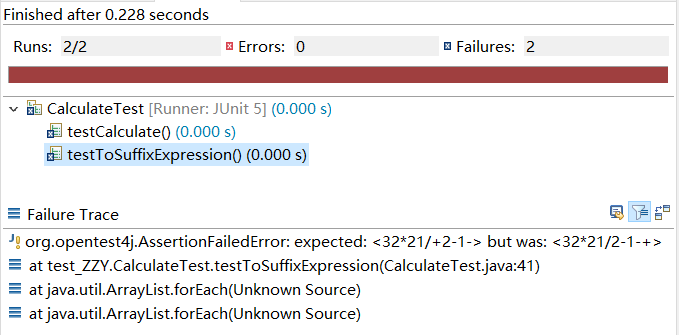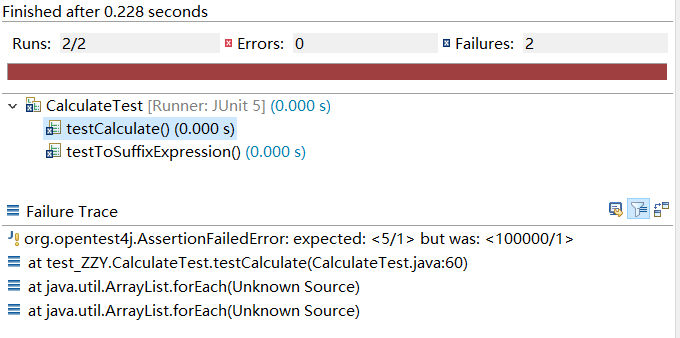• 分析：运算发现，后缀表达式偏差，导致运算过程出现负数，即运算过程违法，所以才导致运算结果不同，实际计算结果一致。Calculate 方法无错，是 ToSuffixExpression 方法的问题。需进一步排查是什么原因导致的表达式偏差。

• 测试用例2 [3 * 2 + 2 / 1 - 2 + 1 =]

• 估计：结果7/1 ，后缀[3, 2, *, 2, 1, / , +,2, -,1, +]

• 实际：结果7/1 ，后缀[3, 2, *, 2, 1, /, 2, -, 1, +, +]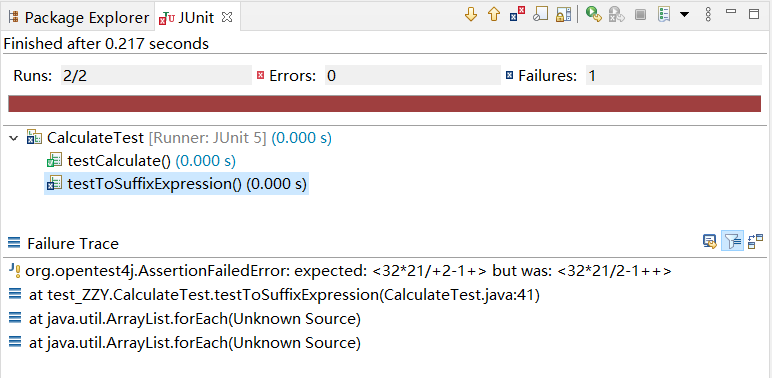• 分析：这一次后缀表达式不同而计算结果一致。仔细推导发现，导致偏差的根源出现在 ToSuffixExpression 方法的符号入栈对比上。入栈对比遇到优先级较高的时，仅仅与前一位符号对比，没有与更之前的进行对比。如此便导致优先级相同时，最左边的反而会沉到后缀表达式的最底下，有一定几率导致 e1-e2 子式中减数与被减数位置对调，出现负数。

• 第二轮测试

• 测试用例1 [3 * 2 + 2 / 1 - 2 - 1 =]

• 估计：结果5/1 ，后缀[3, 2, *, 2, 1, / , +,2, -,1, -]

• 实际：结果5/1 ，后缀[3, 2, *, 2, 1, / , +,2, -,1, -]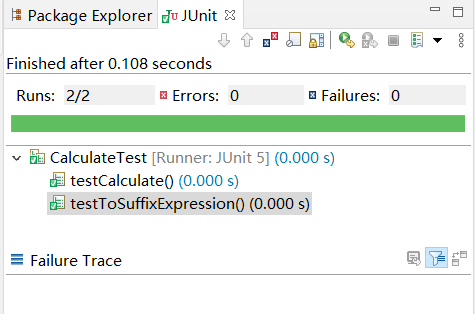• 分析：断言都正确，全部方法均无错

• 测试用例2 [1 + ( 5 - 3 - 1 ) =]

• 估计：结果2/1 ，后缀[1, 5, 3, -, 1, -, +]

• 实际：结果2/1 ，后缀[1, 5, 3, -, 1, -, +]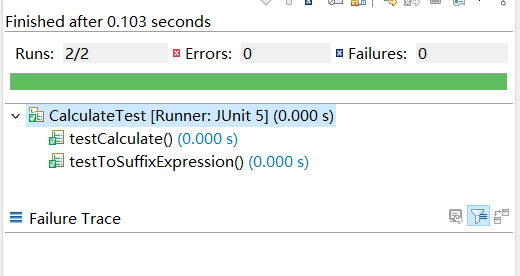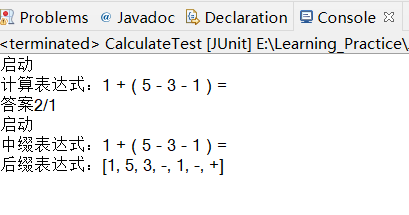• 分析：断言都正确，全部方法均无错

### 2、Expression 类

• 测试方法：
• generateExp 生成原始表达式
• legalExp 生成合法表达式
• 测试
• 结果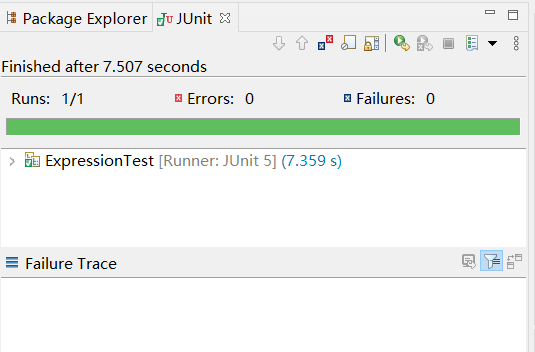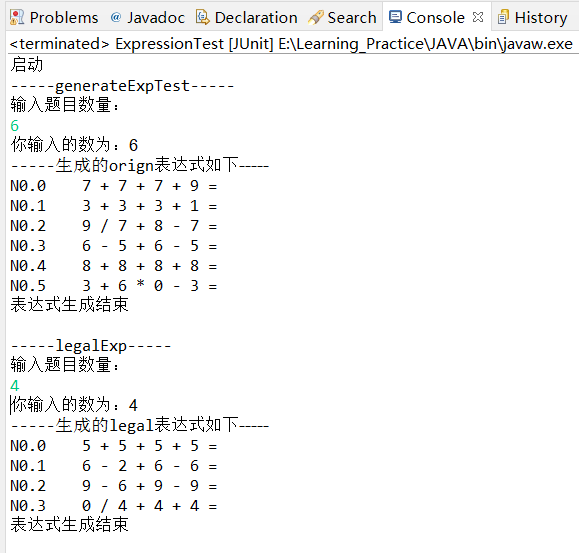• 分析：能根据要求生成指定数量的题目，两个方法均无错

### 2、Fraction 类

• 测试方法：
• add
• sub
• muti
• div
• Appointment 分别约分分子分母
• transferFraction 约分化简，真分数/带分数形式表示
• 测试
• 结果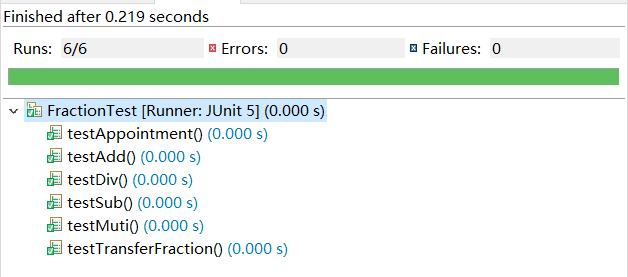• 分析：断言都正确，全部方法均无错

## 四、效能分析

### 具体实现

1. 共花费了20小时。

2. 在实现查重功能时，一开始通过比对生成题目的答案是否和已有的答案进行对比，每次比较，通过遍历由不重复题目的答案组成的字符串数组，因为这样的查重算法的碰撞性较高，限定操作数范围为10以内的数字时，不能生成10000道题目，上限大概为2567道题目，而且效率较低。

3. 在生成题目效率上作了改进，用hashMap代替原有的字符串数组查找是否有相同的结果。调用hashMap中的containsKey(),查看是否有相同的值，不再用for循环一一遍历。虽然效率提高了一点，但是生成题目的上限依然为2567道。

4. 为提高生成题目的上限，改变查重的方法。四则运算用的是中缀转后缀再求值的方法，在用后缀表达式求值时，用字符串记录中间结果，只有中间结果一样才认为题目是重复的。最后可以成功生成一万道题目，上限大概在55778道左右。通过查看生成表达式的方法，发现每一次循环中，运算数和运算符生成时用的同一个随机数，所以让运算数和运算符用不同的随机数，可以提高生成题目的上限，上限大概为239128 道题目，耗时接近23分钟。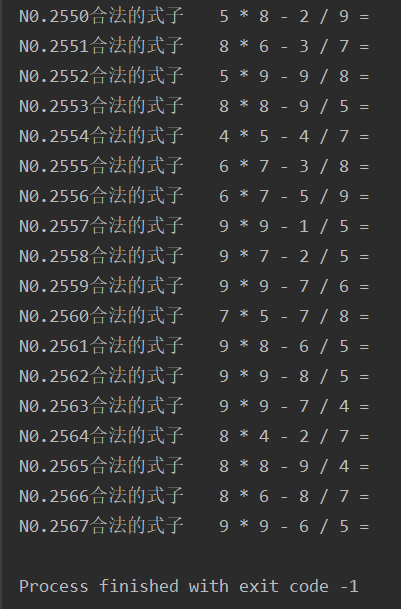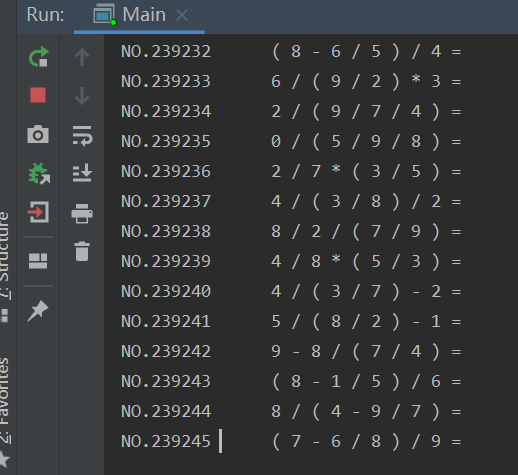### 生成10000道题目时

• 内存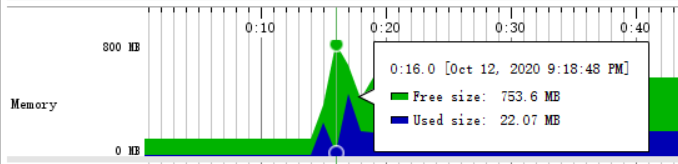• Classes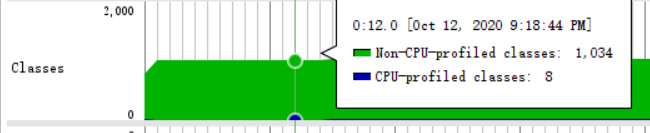• GC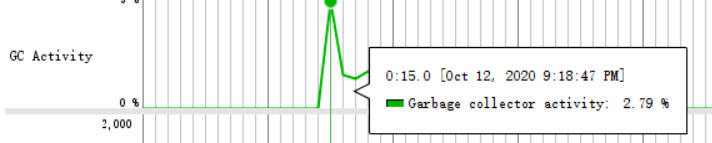• 线程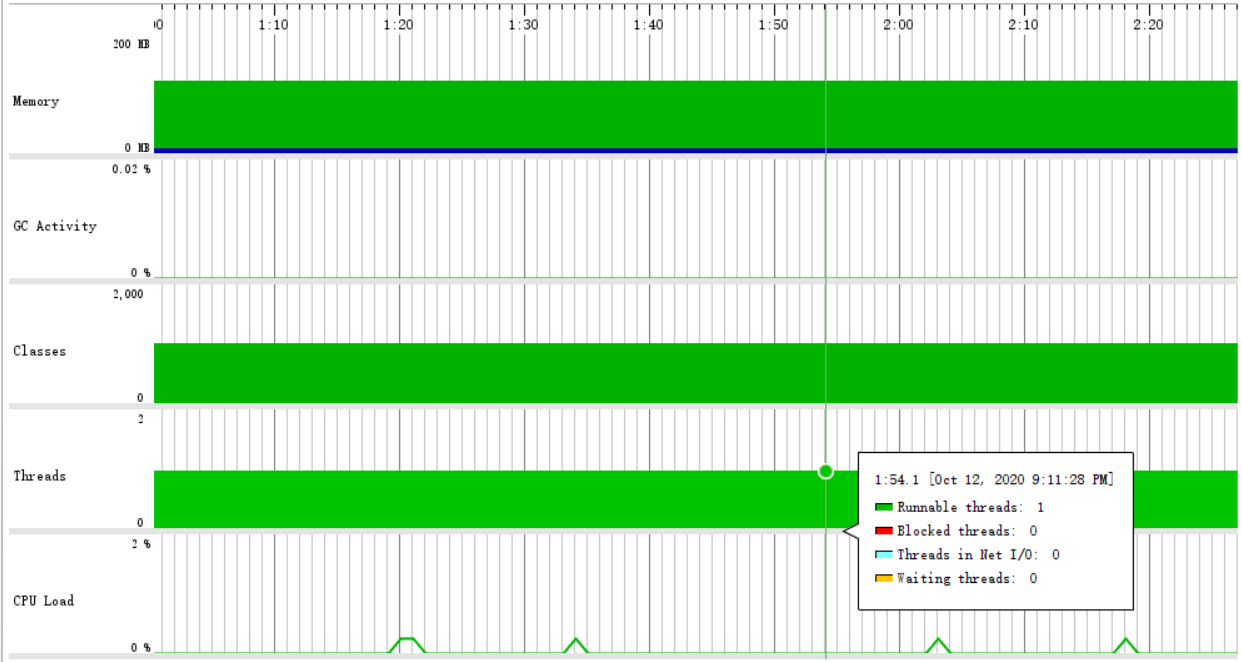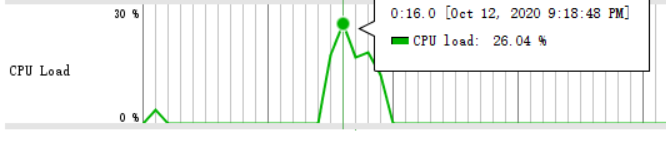## 五、项目小结

#### 李文静

1、收获

2、不足

• 在表示生成时没有考虑真分数的输入，只处理整数。
• 生成括号时，最多只生成一个括号。没有实现生成多个括号的情况。
• 查重时，只对中间结果进行对比，这不是一个很好的方法，有一定的局限性。

3、改进

4、感受

1、收获

2、不足

3、改进

4、结对感受

## 附录 PSP表

PSP2.1 Personal Software Process Stages 预估耗时（分钟） 实际耗时（分钟）
Planning 计划
Estimate 估计这个任务需要多少时间 60 25
Development 开发
Analysis 需求分析 (包括学习新技术) 360 1200
Design Spec 生成设计文档 60 30
Design Review 设计复审 30 10
Coding Standard 代码规范 (为目前的开发制定合适的规范) 30 20
Design 具体设计 120 240
Coding 具体编码 1800 2880
Code Review 代码复审 240 480
Test 测试（自我测试，修改代码，提交修改） 720 600
Reporting 报告
Test Repor 测试报告 120 120
Size Measurement 计算工作量 30 20
Postmortem & Process Improvement Plan 事后总结, 并提出过程改进计划 60 30

posted @ 2020-10-13 01:38  左左左左右  阅读(93)  评论(0编辑  收藏